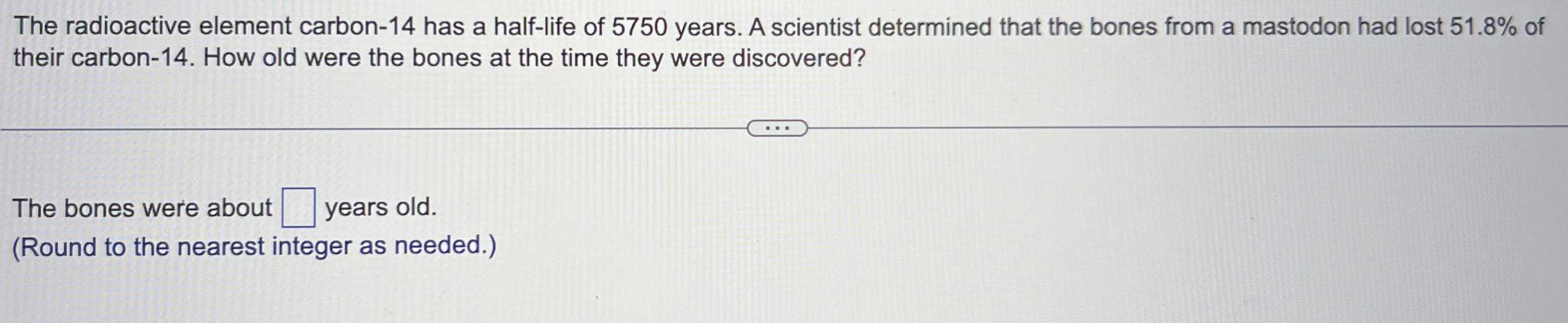### Still have math questions?

Algebra
QuestionThe radioactive element carbon- $$14$$ has a half-life of $$5750$$ years. A scientist determined that the bones from a mastodon had lost $$51.8 \%$$ of their carbon-14. How old were the bones at the time they were discovered?

The bones were about $$\square$$ years old. (Round to the nearest integer as needed.)